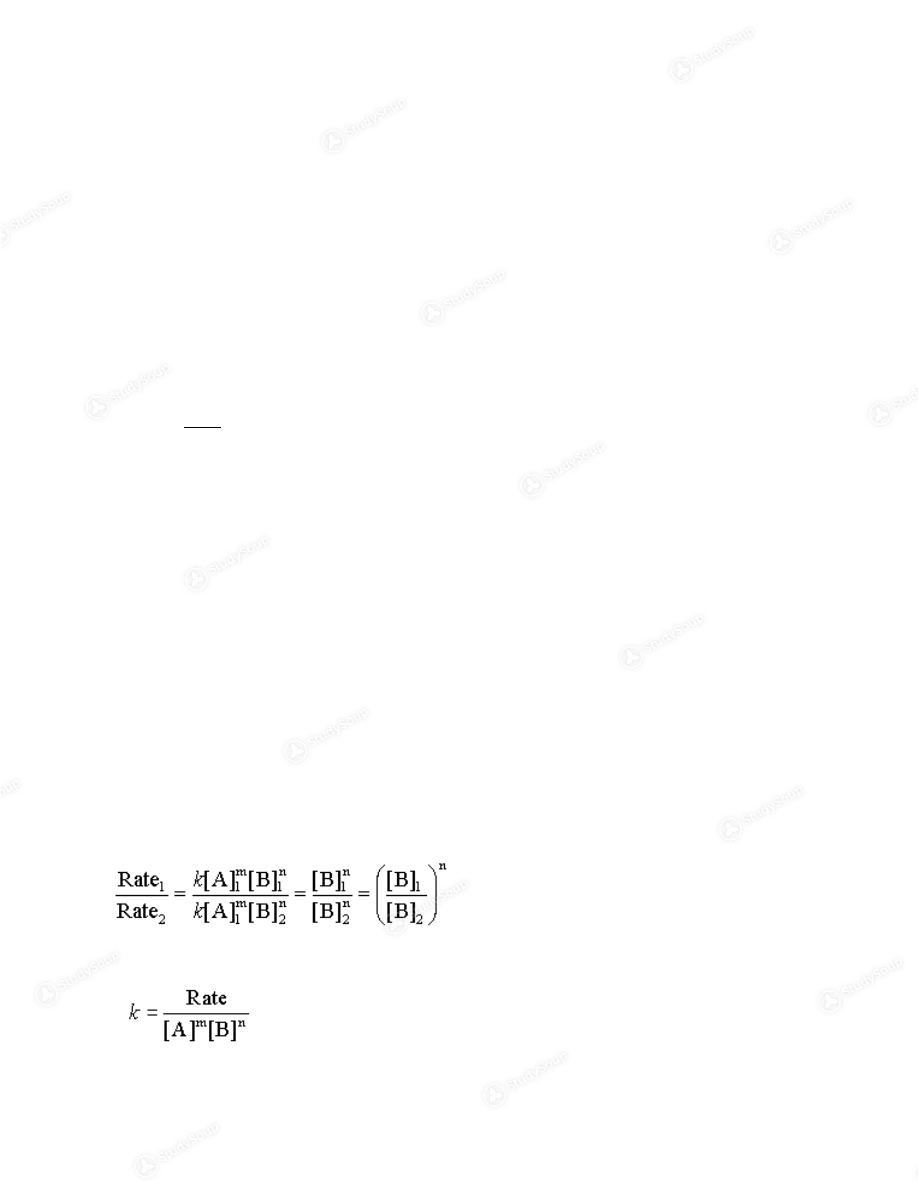Limited time offer 20% OFF StudySoup Subscription details

# URI - CHM 112 - Spring 2018 - CHM 112 Week 1 Notes - Class Notes

### Created by: Emily Fredsbo Elite Notetaker

> > > > URI - CHM 112 - Spring 2018 - CHM 112 Week 1 Notes - Class Notes

URI - CHM 112 - Spring 2018 - CHM 112 Week 1 Notes - Class Notes

##### Description: Rates of Reaction
This preview shows pages 1 - 2 of a 5 page document. to view the rest of the contentCourse Content/Introduction to Kinetics: Chemical Reactivity: How fast do reactions proceed? study of = kinetics To what extent do reactions occur? Can we predict the products of reactions? yes, in some cases What is the relationship between molecular structure and reactivity? What are the energies associated with reactions? Three types of reactivity in aqueous solution: acid/base precipitation oxidation/reduction Rates of Reaction Kinetics is the study of the quantitative aspects of the speed of reactions or rate at which a reaction  occurs Mathematically, this is expressed as: rate = change in concentration/change in time = change in [ ]/change in time = (mol/L)/sec = mol/L/s
=   molL
-1 s -1 ** change in concentration will most likely be molarity (moles per liter) Factors influencing rates: concentration of reactants temperature most speed up with increasing temperature most slow down with decreasing temperature catalysts (materials that change the reaction rate without being consumed in the reaction) make the reaction go faster without changing the stoichiometry inhibitors make the reaction go slower surface area of a solid (either as a reactant or a catalyst)Rates of Reaction: Rate Laws: Consider the general reaction : A + B + C + … -> products
For this reaction: Rate = k[A]
m [B] n [C] p This is called the Rate Law for the reaction k = rate constant m = order of reaction in reactant A n = order of reaction in reactant B p = order of reaction in reactant C The total order of reaction is in the sum of the orders of reaction for each reactant total order = m + n +  p+ … Properties of Rate Laws: orders reaction need not be integers orders of reaction need not be positive catalyst concentrations may be part of a rate law Rate Laws are constant at any given temperature Rate laws  must be found experimentally; there is no information in a balanced reaction about the correct  form of the rate law
Example
2 H 2 (g) + 2NO(g) -> N 2 (g) + 2H 2 O(g) the experimental rate law is found to be Rate = k[H 2 ][NO] 2 What are the orders of reaction in each reactant? What is the total order of reaction? Method of Initial Rates the rate of a reaction is found just at the beginning of the reaction done for several, carefully chosen concentrations of reactants by application of the rate law at each concentration, the orders of reaction and the rate constant can  be found Consider the reaction: A + B -> products Conduct three experiments [A] 1 ,[B] 1 , measure Rate  1 [A] 1 ,[B] 2 , measure Rate  2 [A] 2 ,[B] 1 , measure Rate  3 From the definition of a rate law: Rate  1  = k[A] 1 m [B] 1 n Rate  2  = k[A] 1 m [B] 2 n Divide the two rates: Rate  1,  Rate  2,  [B] 1,  [B] are known so simple algebra gives the order of reaction in reactant B Similarly for reactant A So the order in reactant A is found Finally, the rate constant is found by using any (or, better, all) of the experiments and the wales of m and  n just determined

This is the end of the preview. Please to view the rest of the contentJoin more than 18,000+ college students at University of Rhode Island who use StudySoup to get ahead
5 Pages 28 Views 22 Unlocks
• Notes, Study Guides, Flashcards + More!Join more than 18,000+ college students at University of Rhode Island who use StudySoup to get ahead
##### Description: Rates of Reaction
5 Pages 28 Views 22 Unlocks
• Notes, Study Guides, Flashcards + More!
Get Full Access to URI - CHM 112 - Class Notes - Week 1
×
Get Full Access to URI - CHM 112 - Class Notes - Week 1

I don't want to reset my password

Need help? Contact support

Need an Account? Is not associated with an account
We're here to help